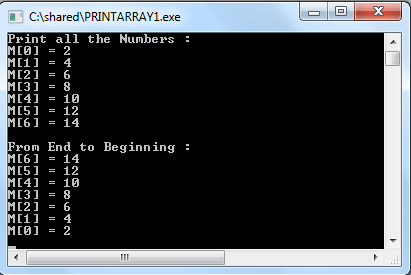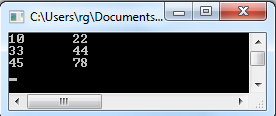## Description

Suppose we want to store 50 student names of a class. One might try to declare 50 variables, say st_name0, st_name1,..,st_name49. However, it is much better to deÃ¯Â¬Âne all those names in a single variable, which is called an array.

- An array is a collection of data of the same type (and therefore, the same size) stored in consecutive memory cells under one name.

- An entire array be declared all at once.

- Each individual element in the array can be referenced by indexing. Indexed means the array elements are numbered and always start at 0.

In C, an element of an array (i.e., an individual data item) is referred to by specifying the array name followed by one or more subscripts, with each subscript enclosed in square brackets.

## Declaring an Array

An array declaration is similar to the form of a normal declaration. The general form is :

typeName ArrayName[size] = { list of values }

This declares an array with the specified size, named ArrayName and type typeName. The array is indexed from 0 to (size-1). The size (in brackets) must be an integer literal or a constant variable. The compiler uses the size to determine how much space to allocate (i.e. how many bytes). Here are some example :

int A; // An array of ten integers; A, A, …, A

char name; // An array of 20 characters

float nums; // An array of fifty floating numbers; nums, nums, …,nums

int C[]; // An array of an unknown number of integers; C, C, …, C[size-1]

int table; // A two dimensional array of integers

You can also declare an array in the following way :

#define ROLL_NUMBERS 51
int classv[ROLL_NUMBERS];

const int Emp_salary = 35
float Employee[Emp_salary];

## Example: int A;

• Declares 10 integers and can be accessed by the name A
• Each variable is assigned a unique location (location is also called as index). The range of the location is 0 to (length – 1). For the said array range of the location is 0 to 9.

See the following diagram which represent the array A :

 Index 0 1 2 3 4 5 6 7 8 9 Value 15 8 14 45 48 45 -57 45 1 0

## Indexing into Arrays

To refer a single array element use arrayname[index].

For example :

A contains the value 48

A contains the value 0

Indexed elements can be used just like simple variables.

printf(“%d\n”, A); // print 48

## Array elements and loops

Array elements are commonly used in for loops, see the following codes :

for(x=0; x < max; x++)
A[x] = x*x;

sum = 0;
for(x=0; x < max; x++)
sum += B[x];

## Array Initialization

We declare normal variables in the following ways :

int x;
x = 0;
or
int x = 0;

In case of array, simply list the array values in set notation { }. Some valid array declarations are shown below.

int num = {1, 3, 5, 7, 9, 11};
char letters = {'a', 'b', 'c', 'd', 'e'};
float numbers = {13.25, 12.09, 8.1};

## Using Array Elements in Expressions

Array elements may be used wherever a variable of the same type may be used. See the following diagram and codes.

Example: int A;

 Index 0 1 2 3 4 5 6 7 8 9 value 15 8 14 45 48 45 -57 45 1 0

x = A * 2;     /* sets x to 16 */

A = 88;    /* replaces 48 with 88 */

m = 6;

y = A[m];   /* sets y to –57 */

z = A[A]; /* sets z to 15 */

## Example -1 :

There are seven numbers store in an array. The following program prints all the numbers of that array as well as prints the numbers in backwards.

1. #include<stdio.h><br>
2. int main()
3. {
4. int M;
5. int j;
6. /* store seven numbers in array M */
7.  M = 2;
8.  M = 4;
9.  M = 6;
10.  M = 8;
11.  M = 10;
12.  M = 12;
13.  M = 14;
14. /* print numbers in M */
15.  printf("Print all the Numbers : \n");
16.  for (j = 0; j < 7; ++j)
17.  printf("M[%d] = %d\n",j,M[j]);
18. /* print numbers in M backwards */<br> printf("\nFrom End to Beginning : \n");
19.  for (j = 6; j >= 0; --j)
20.  {
21.  printf("M[%d] = %d\n",j,M[j]);
22.   }
23. }

## Output## Example -2 :

The following program compute prime numbers between 1 to MXNO.

1. #include<stdio.h>
2.   #define MXNO 50
3. int main()
4. {
5.   int M[MXNO];
6.   int j, n;
7.  /* mark all numbers */
8.   printf("The prime numbers are :\n\n");
9.   for (j = 0; j < MXNO; ++j)
10.   {
11.   M[j] = 1;
12.   }
13.   for (j = 2; j < MXNO; ++j)
14.   {
15.   if (M[j])
16.   {
17.   printf("%d\n", j);
18.   }
19.   for (n = j+j; n < MXNO; n+= j)
20.   {
21.   M[n] = 0;
22.   }
23.   }
24. }

## Copying arrays:

We have two arrays list1 and list2

int list1 = {2, 4, 6, 8, 10, 12};

int list2;

and we want to copy the contents of list1 to list2. For general variables (e.g. int x=3, y=5) we use simple assignment statement (x=y or y=x). But for arrays the following statement is wrong.

list2 = list1;

We must copy between arrays element by element and the two arrays must have the same size. In the following example we use a for loop which make this easy.

1. #include <stdio.h>
2. main()
3. int list1 = {2, 4, 6, 8, 10, 12};
4. int list2;
5. for (int ctr = 0; ctr<6; ctr++)
6. {
7.  list2[ctr] = list1[ctr];
8. }
9.  printf("Elements of list2 :\n");
10.  for (int ctr = 0; ctr<6; ctr++)
11.  {
12.   printf("%d ",list2[ctr]);
13.  }
14. }

## Output

Elements of list2 :

2 4 6 8 10 12

## Two dimensional Array in C

A two-dimensional array can be used to represent a matrix, a table or board games (Tic Tac Toe, Sudoku etc). The row and column positions are given as successive indices. When you declare a variable of such an array, use a pair of square brackets for each dimension. See the following example :

// Declare a two dimensional array with 3 rows and 2 columns

int table:

// create and initialize an array

int table = { {10, 22}, {33, 44}, {45, 78} };

or

int table = {10, 22, 33, 44, 45, 78 };

or

int table = {

{10, 22},

{33, 44 },

{45, 78 }

};

See the following diagram which represents the above array :

 0 1 0 10 22 1 33 44 2 45 78

In the above array :

table contains 22

table contains 45

table is “array out of bounds”

## Printing a Two dimensional array

To print all the elements of a two dimension array we use a doubly-nested for-loop. In the following example there are 3 rows and 2 columns.

1. #include <stdio.h>
2. main()
3. {
4.   int row,col;
5.   int table = { {10, 22}, {33, 44}, {45, 78} };
6.   for (row = 0; row < 3; row++)
7.   {
8.   for (col = 0; col < 2; col++)
9.   {
10.   printf("%d\t",table[row][col]);
11.   }
12.   printf("\n");
13.   }
14. }

## Outputsource:http://www.w3resource.com/c-programming/c-array.php

Ã¯Â»Â¿